# Texas Go Math Kindergarten Lesson 10.4 Answer Key Compose 10

Refer to our Texas Go Math Kindergarten Answer Key Pdf to score good marks in the exams. Test yourself by practicing the problems from Texas Go Math Kindergarten Lesson 10.4 Answer Key Compose 10.

## Texas Go Math Kindergarten Lesson 10.4 Answer Key Compose 10

Essential Question
How do you put together numbers to make 10?

Explore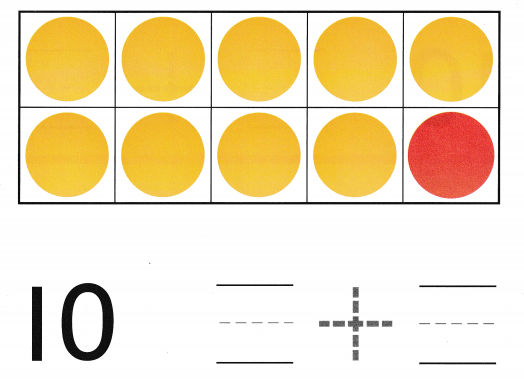Explanation:
The number of yellow counters are 9
The number of Red counters are 1
The total number of  Yellow and Red counters are 10
Since 9 + 1 = 10

Directions
Place yellow and red counters in the ten frame as shown. Write the numbers and trace the symbol to show the number pair of 10.

Share and Show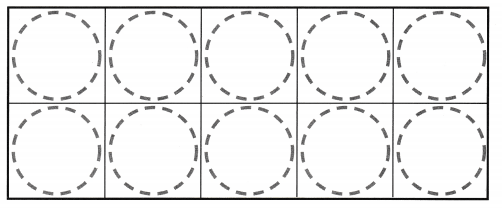Question 1.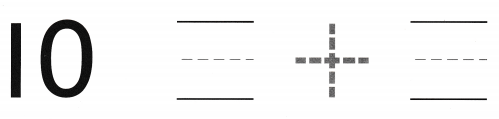Explanation:
The number of yellow counters are 8
The number of Red counters are 2
The total number of  Yellow and Red counters are 10
Since 8 + 2 = 10

Question 2.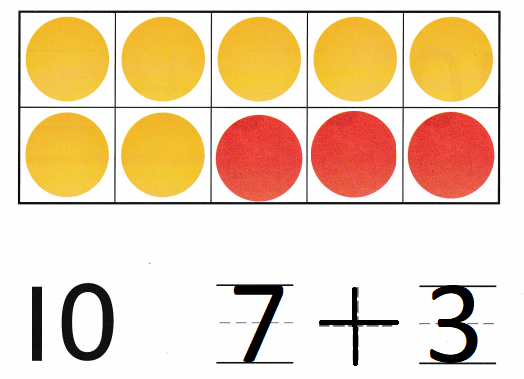Explanation:
The number of yellow counters are 7
The number of Red counters are 3
The total number of  Yellow and Red counters are 10
Since 7 + 3 = 10

Question 3.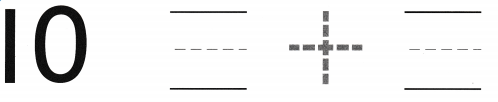Explanation:
The number of yellow counters are 6
The number of Red counters are 4
The total number of  Yellow and Red counters are 10
Since 6 + 4 = 10

Directions
Use counters to show number pairs that make 10. 1-3. Write the number pair and trace the symbol. For Exercise 3, color the counters to model the number pair that makes 10.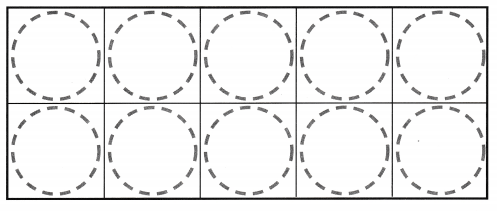Question 4.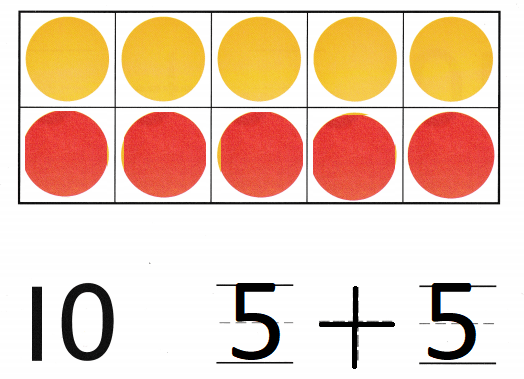Explanation:
The number of yellow counters are 5
The number of Red counters are 5
The total number of  Yellow and Red counters are 10
Since 5 + 5 = 10

Question 5.Explanation:
The number of yellow counters are 4
The number of Red counters are 6
The total number of  Yellow and Red counters are 10
Since 4 + 6 = 10

Question 6.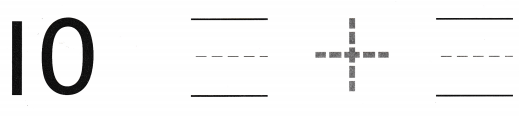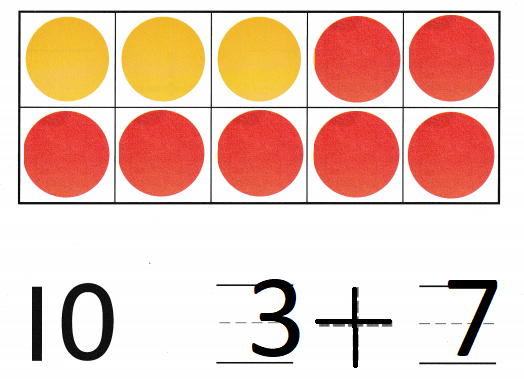Explanation:
The number of yellow counters are 3
The number of Red counters are 7
The total number of  Yellow and Red counters are 10
Since 3 + 7 = 10

Directions
Use counters to show number pairs that make 10. 4-6. Write the number pair and trace the symbol. For Exercise 6. color the counters to model the number pair that makes 10.

Home Activity

• Have your child use his or her fingers on two hands to show a number pair for 10.

Problem Solving

Question 7.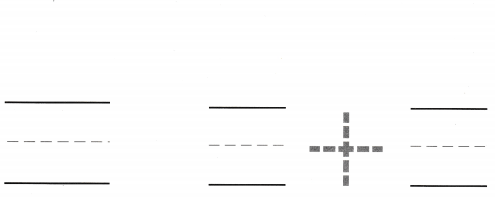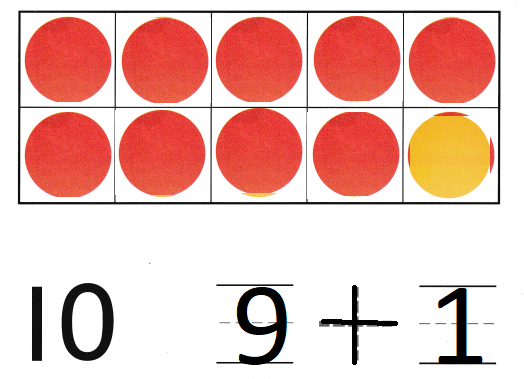Explanation:
The number of Red counters are 9
The number of yellow counters are 1
The total number of  Yellow and Red counters are 10
Since 9 + 1 = 10

Question 8.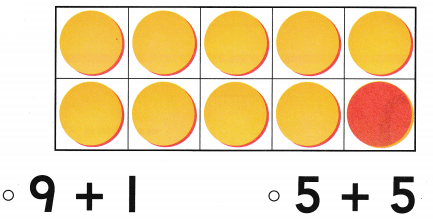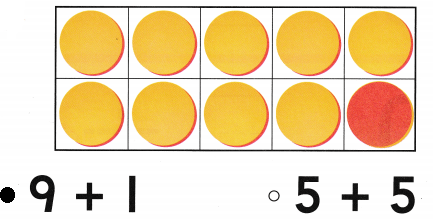Explanation:
The number of yellow counters are 9
The number of Red counters are 1
The total number of  Yellow and Red counters are 10
Since 9 + 1 = 10

Directions
7. Riley has 9 red counters and 1 yellow counter. How many counters does she have? Draw to solve the problem. Write the numbers and trace the symbol. 8. Choose the correct answer Count the counters, Which number pair is shown?

### Texas Go Math Kindergarten Lesson 10.4 Homework and Practice

Question 1.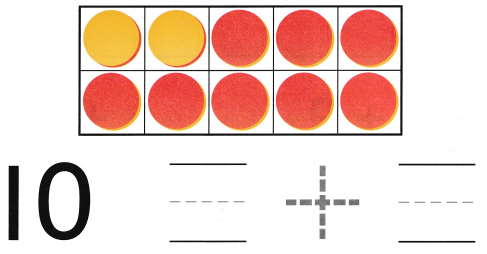Explanation:
The number of yellow counters are 2
The number of Red counters are 8
The total number of  Yellow and Red counters are 10
Since 2 + 8 = 10
Question 2.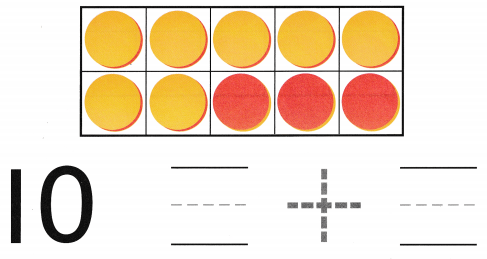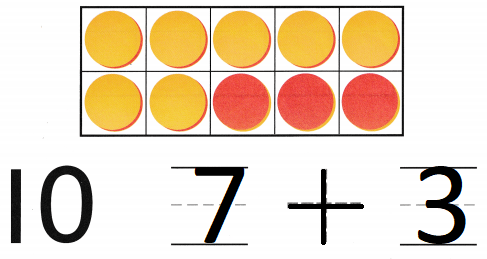Explanation:
The number of yellow counters are 7
The number of Red counters are 3
The total number of  Yellow and Red counters are 10
Since 7 + 3 = 10

Directions
1-2. Count the counters in the ten frame that model the number pair that makes 10. Write the number pair and trace the symbol.

Texas Test Prep

Lesson Check

Question 3.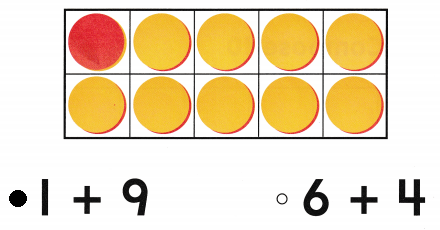Explanation:
The number of Red counters are 1
The number of yellow counters are 9
The total number of  Yellow and Red counters are 10
Since 1 + 9 = 10

Question 4.